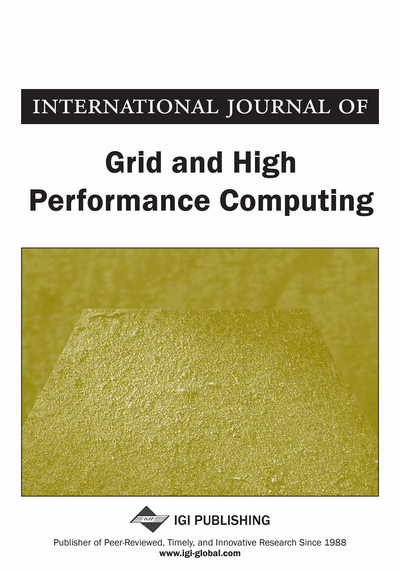# High Performance CGM-based Parallel Algorithms for the Optimal Binary Search Tree Problem

Vianney Kengne Tchendji (Department of Mathematics and Computer Science, University of Dschang, Dschang, Cameroon), Jean Frederic Myoupo (University of Picardie Jules Verne, Amiens, France) and Gilles Dequen (University of Picardie Jules Verne, Amiens, France)
DOI: 10.4018/IJGHPC.2016100104

## Abstract

In this paper, the authors highlight the existence of close relations between the execution time, efficiency and number of communication rounds in a family of CGM-based parallel algorithms for the optimal binary search tree problem (OBST). In this case, these three parameters cannot be simultaneously improved. The family of CGM (Coarse Grained Multicomputer) algorithms they derive is based on Knuth's sequential solution running in time and space, where n is the size of the problem. These CGM algorithms use p processors, each with local memory. In general, the authors show that each algorithms runs in with communications rounds. is the granularity of their model, and is a parameter that depends on and . The special case of yields a load-balanced CGM-based parallel algorithm with communication rounds and execution steps. Alternately, if , they obtain another algorithm with better execution time, say , the absence of any load-balancing and communication rounds, i.e., not better than the first algorithm. The authors show that the granularity has a crucial role in the different techniques they use to partition the problem to solve and study the impact of each scheduling algorithm. To the best of their knowledge, this is the first unified method to derive a set of parameter-dependent CGM-based parallel algorithms for the OBST problem.
Article Preview
Top

## 1. Introduction

The Optimal Binary Search Tree (OBST) problem is of great interest in data processing, as well as in distributed and centralized environments. The input is a sequence of n weighted keys (k1, k2, …, kn), which are to be placed into a binary search tree in such a manner that the expected search cost of the resulting binary search tree is minimized (Cormen et al., 2001). The classical sequential algorithm for this problem is based on the dynamic programming technique (Aho et al., 1974). It requirestime steps andmemory space. By using the monotonicity property of optimal binary search trees (defined later), Knuth (1972) derived antime steps algorithm in the same space. Yao (1982) obtained the same result thanks to the quadrangle inequalities. Eppstein et al. (1988) put forth analgorithm using the restrictive assumption of convexity, whereas the parallelization of the classical version was extensively utilized by the community of parallel processing researchers for different parallel computing models: Bradford (1994), Guibas, Kung and Thompson (1979), Tang and Gupta (1995), Karypis et al. (1994, 1996), Rytter (1988), and Kechid and Myoupo (2008). Little work has been produced for the parallelization of the Knuth approach. An efficient parallelization of this version in an abstract model, such as PRAM (Parallel Random Access Machine), is difficult. In PRAM, it is unknown how to use the monotonicity property to reduce the number of processors in poly-logarithmic-time computations (Karpinski et al., 1994, 1996).

In this work, we tackle the problem of parallelizing the OBST algorithm on the Bridging Coarse Grain BSP/CGM (Bulk Synchronous Parallel/Coarse Grained Multicomputer) model (Dehne et al., 1994, 1996; Cáceres et al., 1997; Cheatham et al., 1995; Valiant, 1990). CGM seems best suited for the design of algorithms that are not too dependent on an individual architecture. A BSP/CGM machine is a set of p processors, each having its own local memory of size m (with O(m)>>O(1)) and connected to a router able to deliver messages in point-to-point fashion. A BSP/CGM algorithm consists of alternating between local computations and global communication rounds. Each communication round consists of routing a single h-relation with h=O(m). A CGM computation/communication round corresponds to a BSP super-step with communication cost(Bradford, 1994).is the cost of the communication of a word in the BSP model. To produce an efficient BSP/CGM algorithm, designers tend to maximize speedup and minimize the number of communication rounds (ideally independent from the problem size, and constant in the optimum case).

## Complete Article List

Search this Journal:
Reset
Open Access Articles: Forthcoming
Volume 12: 4 Issues (2020): 2 Released, 2 Forthcoming
Volume 11: 4 Issues (2019)
Volume 10: 4 Issues (2018)
Volume 9: 4 Issues (2017)
Volume 8: 4 Issues (2016)
Volume 7: 4 Issues (2015)
Volume 6: 4 Issues (2014)
Volume 5: 4 Issues (2013)
Volume 4: 4 Issues (2012)
Volume 3: 4 Issues (2011)
Volume 2: 4 Issues (2010)
Volume 1: 4 Issues (2009)
View Complete Journal Contents Listing